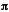home | O'Reilly's CD bookshelfs | FreeBSD | Linux | Cisco | Cisco Exam## 2.11. Doing Trigonometry in Degrees, not Radians

### Solution

Convert between radians and degrees (2radians equals 360 degrees).

```BEGIN {
use constant PI => 3.14159265358979;

my \$degrees = shift;
return (\$degrees / 180) * PI;
}

return (\$radians / PI) * 180;
}
}```

Alternatively, use the Math::Trig module.

```use Math::Trig;

### Discussion

If you're doing a lot of trigonometry, look into using either the standard Math::Trig or POSIX modules. They provide many more trigonometric functions than are defined in the Perl core. Otherwise, the first solution above will define the ``` rad2deg``` and ``` deg2rad``` functions. The value ofisn't built directly into Perl, but you can calculate it to as much precision as your floating-point hardware provides. If you put it in a BEGIN block, this is done at compile time. In the solution above, the ``` PI``` function is a constant created with ``` use``` ``` constant``` .

If you're looking for the sine in degrees, use this:

```# deg2rad and rad2deg defined either as above or from Math::Trig
sub degree_sine {
my \$degrees = shift;

return \$result;
}```

The ``` sin``` , ``` cos``` , and ``` atan2``` functions in perlfunc (1) and Chapter 3 of Programming Perl ; the documentation for the standard POSIX and Math::Trig modules (also in Chapter 7 of Programming Perl )2.10. Generating Biased Random Numbers2.12. Calculating More Trigonometric Functions